# Conformal Models of the Hyperbolic Geometry

Vladimir Bulatov
Corvallis, Oregon, USA
http://bulatov.org
• Disk Model of Hyperbolic Plane H2
• Upper Half Plane Model of H2
• General Moebius Map Model of H2
• Band Model of H2
• Special Orientations of Band Model
• Mixing Fixed Points
• Generalizations of Band Model
• Fancy Conformal Mappings
• Generalization to H3

Presented at MAA-AMS Joint Mathematics Meeting. San Francisco, January 17, 2010

Use arrows or PgUp/PgDown keys to navigate between the slides. GIF animations opens in a new window. Works best with Firefox.

# Poincare (Disk) Model of H2Poincare disk model of hyperbolic plane. Geodesics in this model are segments of circles orthogonal to the unit circle (circle at infinity). The model is conformal - the angles between intersecting geodesics are equal to the euclidean angles between the tangent lines of the circle. To visualize the hyperbolic geometry we will be using this tiling with right angled regular pentagons. It is analog of simple square tiling of the euclidean plane.

# Pentagons ARE RegularBy moving the center of a pentagon into the center of Poincare circle we can see that pentagons are actually regular.

# Rotation of H2 around axis of 4th order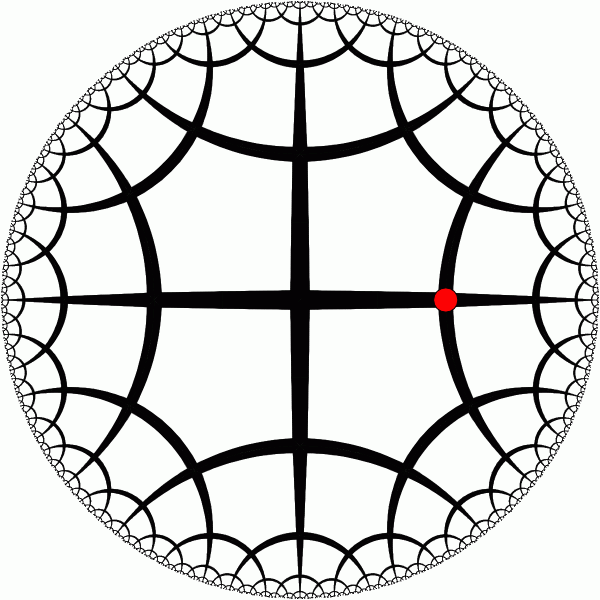Hyperbolic plane has isometries similar to the isometries of the euclidean plane. Here we have rotation around symmetry axis of 4th order. [animation]

# Translation of H2 along X-axis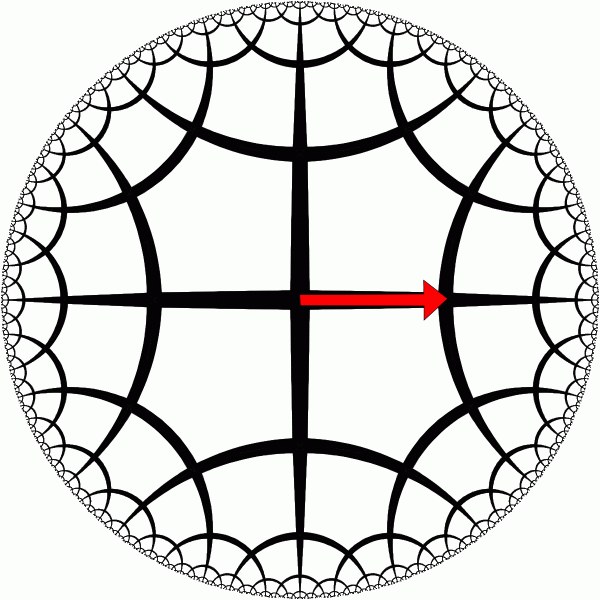Hyperbolic translation along X-axis. [animation]

# Ideal Rotation of H2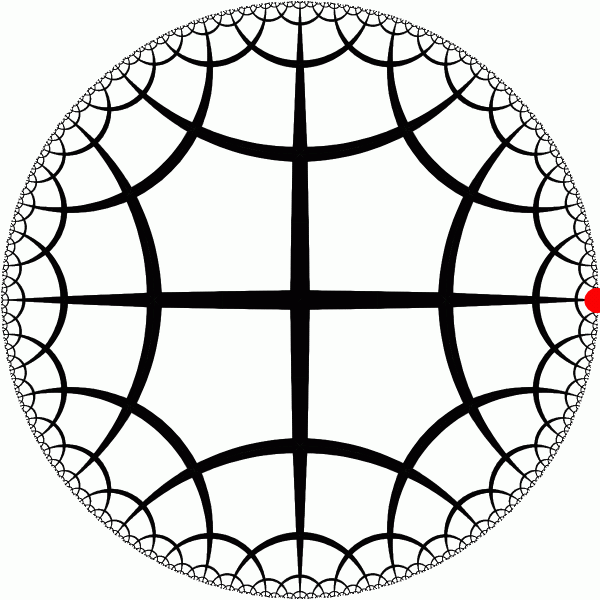This ideal rotation around the point at infinity has no analog in the euclidean geometry. [animation]

# Upper Half Plane Model of H2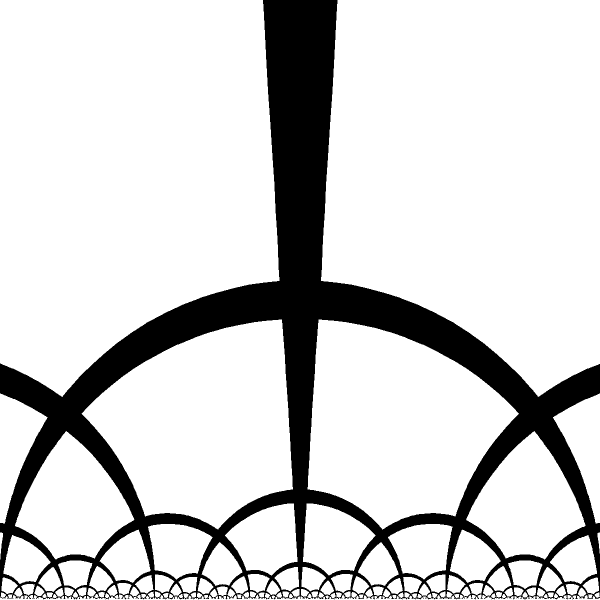Upper half plane model is another standard model of the hyperbolic geometry. Geodesics are half circles orthogonal to the horizontal axis. The model is also conformal.

# Rotation in the Upper Half Plane Model

 m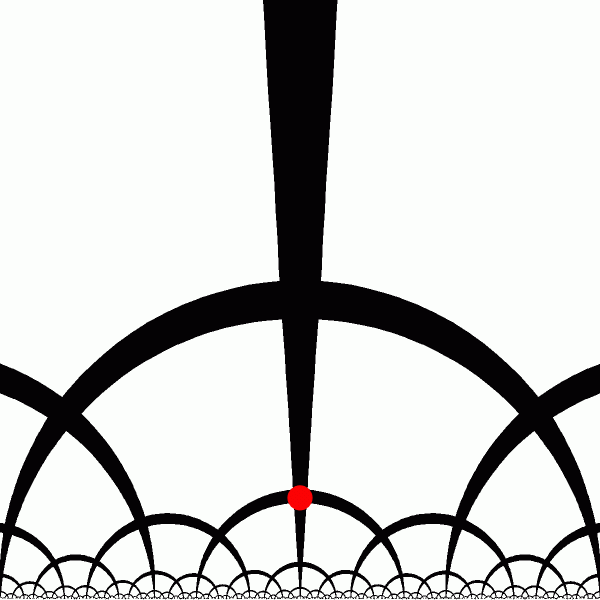This is a rotation of the hyperbolic plane around an axis of 4th order [animation]

# Translation in the Upper Half Plane Model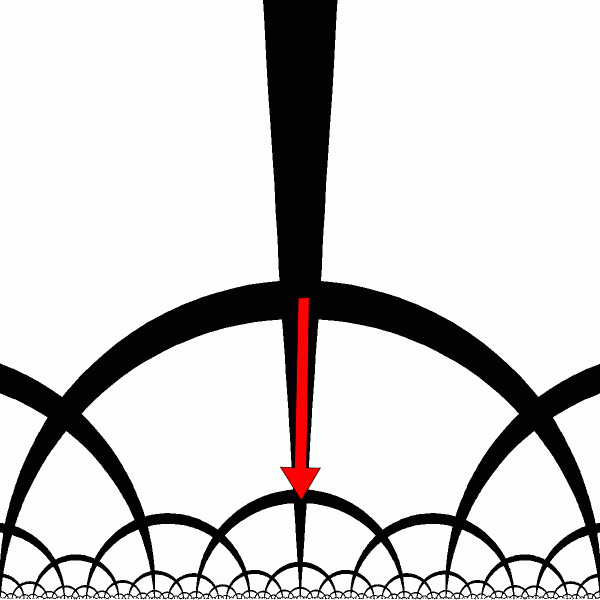This is translation of the hyperbolic plane in Y direction in the upper half plane model [animation]

# Ideal Rotation of the Upper Half Plane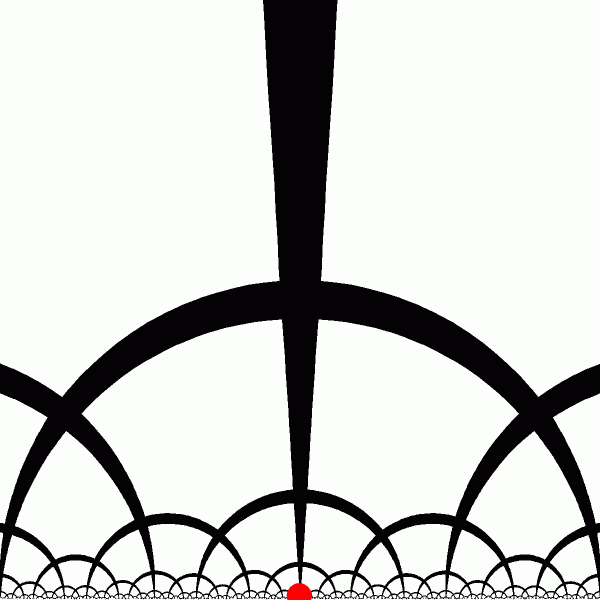Ideal Rotation around a point at infinity [animation]

# Moebius Transformation of the DiskIsometries of the disk model and the upper half plane models are particular cases of the Moebius transformation - general linear fractional transformation of the complex plane $C$: M(z) = (az+b)/(cz+d) where a, b, c, d, z in CC, ad-bc=1 This transformation can also transform the disk model into the upper half plane model and into everything in between. [animation]

# Band Model of H2Band model of the hyperbolic plane is obtained by applying to the disk model a special conformal map of the complex plane: w =(2/\pi)tanh\^-1(z); z, w \in CC, |Im(w)| < 1, |z| < 1 Particular points are mapped as follows: [[1,\to,+\infty],[-1,\to,-\infty],[i,\to,i],[-i,\to,-i]] The band model is conformal, because it is obtained from disk model using conformal transformation. [animation]

# Translation of H2 along X axis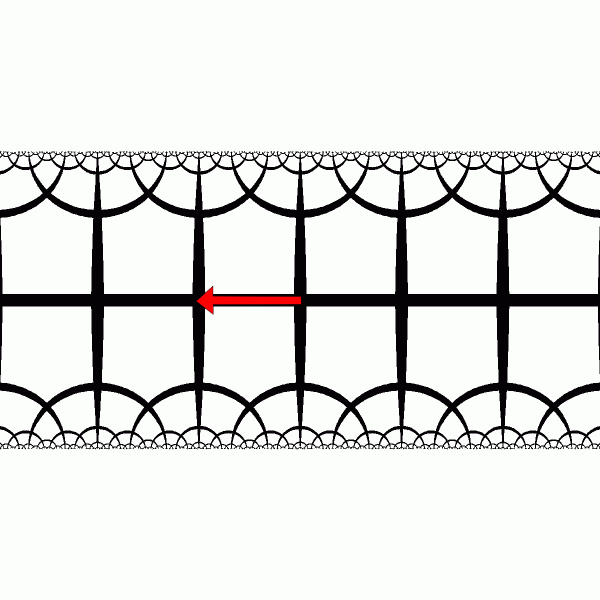Hyperbolic metric in the band model is given by: (2/\pi)|dz|/ cos(Im(z)) [Hubbard J. - Teichmüller Theory.] At the center line of the band $|Im(z)| = 0$ the metric is euclidean. X-translation of the hyperbolic plane is simple euclidean translation. [animation]

# Translation of H2 along Y axis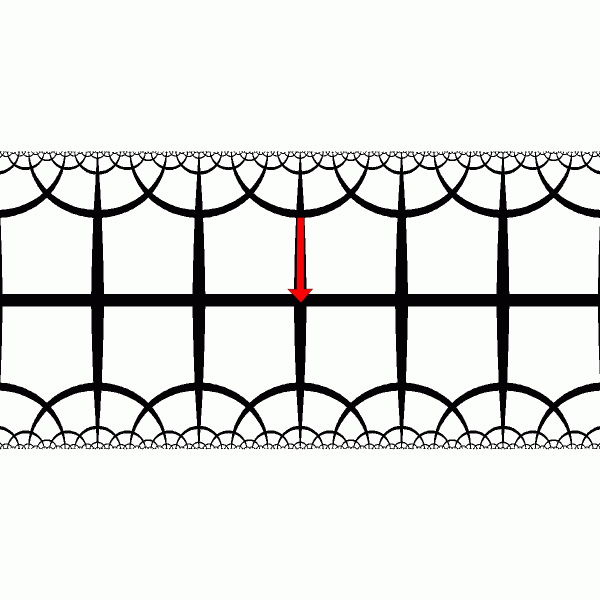Y - translation looks more complex. [animation]

# Rotation of H2 in the Band model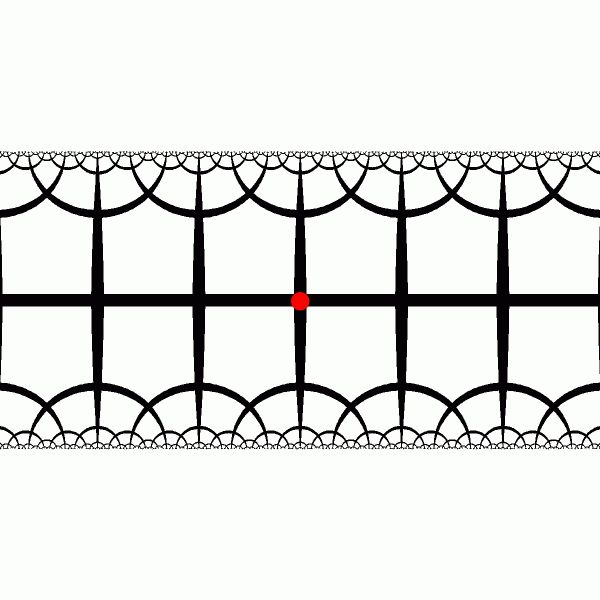Rotation in band model looks very accelerated at the ends of the band [animation]

# Ideal Rotation in the Band Model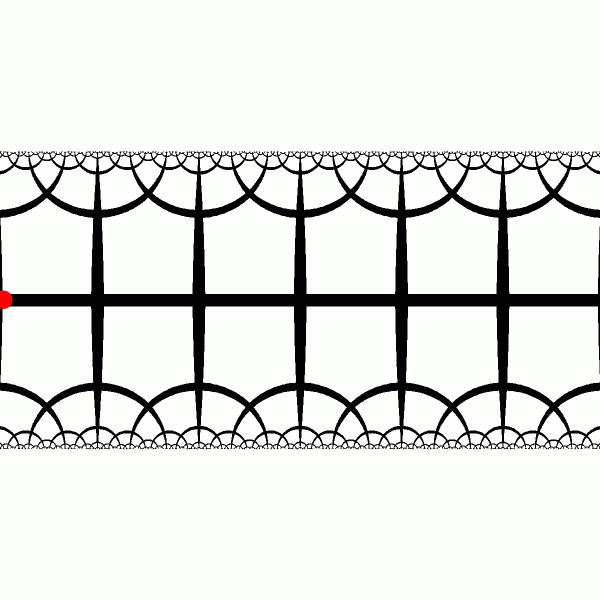Ideal rotation around a point at $-\infty$ significanly moves only right half of the band. [animation]

# Special Orientation of H2 in Band Model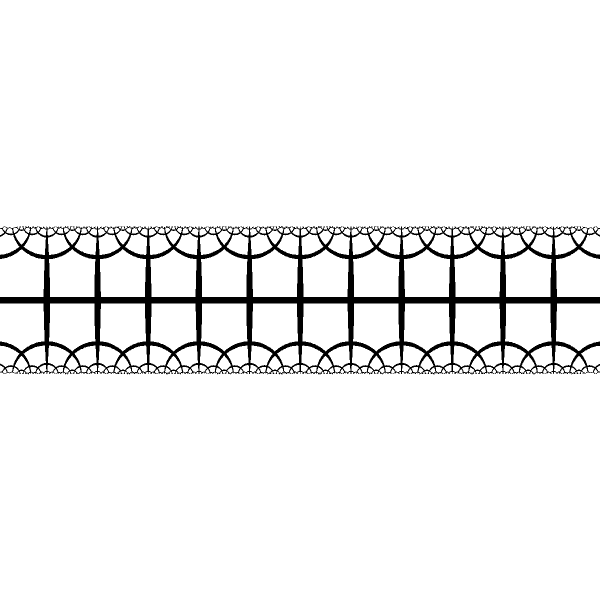The hyperbolic tiling in the band model is periodic in R2. Is this coincidence?

# Random Transformation of H2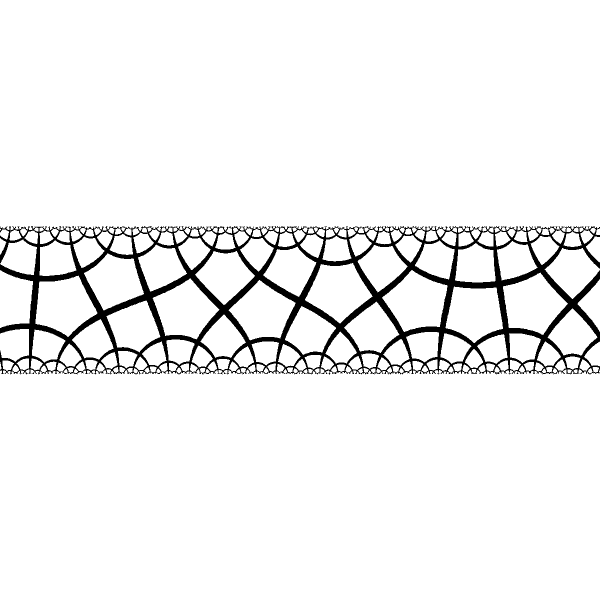In the general case the tilng will not be peiodical in R2. In this case no period is visible.

# Fixed Points of Moebius Transformation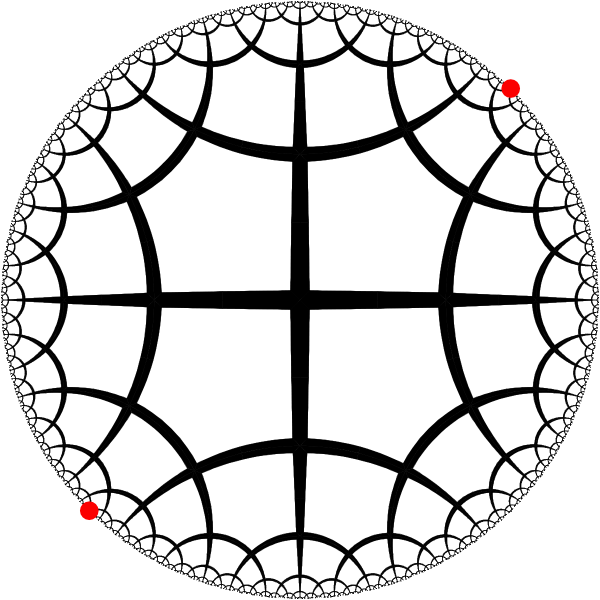Symmetry transformations of the hyperbolic tiling are isometries of the hyperbolic plane. Isometries of the disk model are Moebius transformations with one or 2 fixed points. Transfomations with a pair of fixed points on the boundary of the unit disk correspond to some translation.

# Canonical Orientation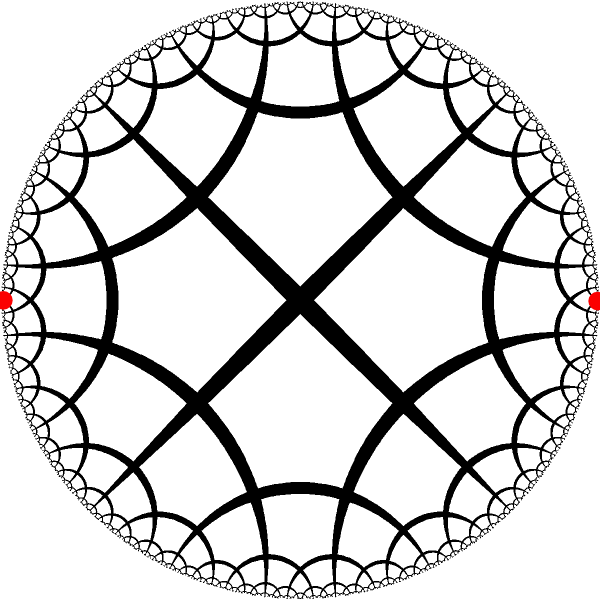We can use isometry of H2 to move the pair of selected fixed points into the canonical positions $-1$ and $1$

# Stretch Disk to BandAfter applying tanh^-1 transformation to the disk we have the band. The tiling now is periodic, though its period is different and it looks differently.# Different Periods in the Band Model 1Period varies for different pairs of fixed points.

# Different Periods in the Band Model 2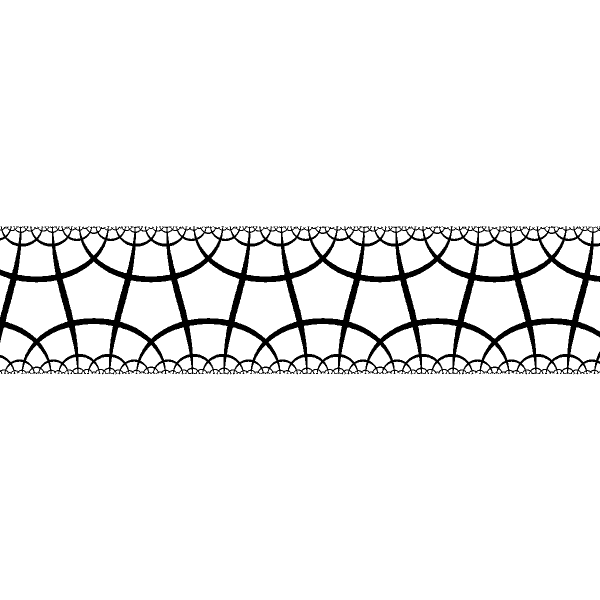Period varies for different pairs of fixed points.

# Different Periods in the Band Model 3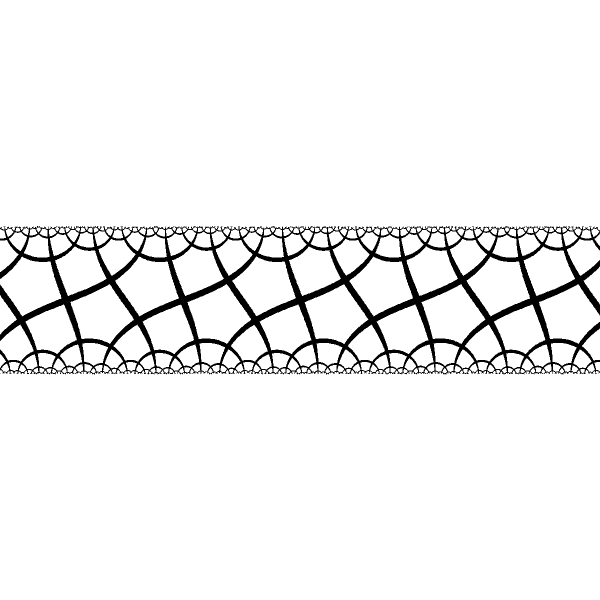Period varies for different pairs of fixed points.

# Different Periods in the Band Model 4Period varies for different pairs of fixed points.

# Different Periods in the Band Model 5Period varies for different pairs of fixed points.

# Different Periods in the Band Model 6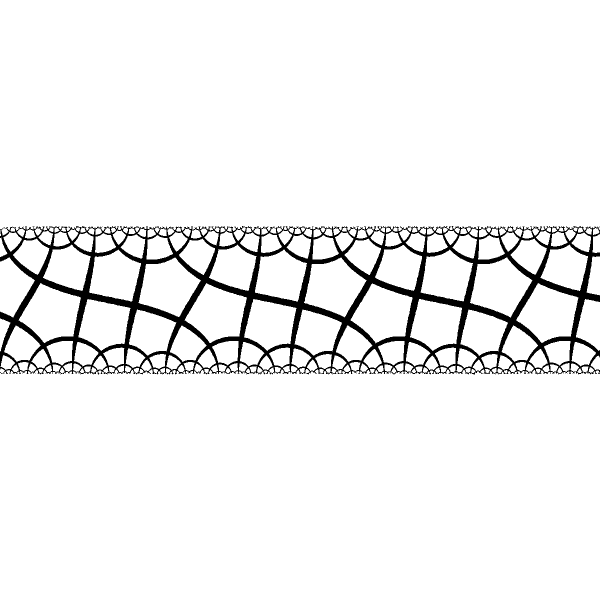Period varies for different pairs of fixed points.

# Different Periods in the Band Model 7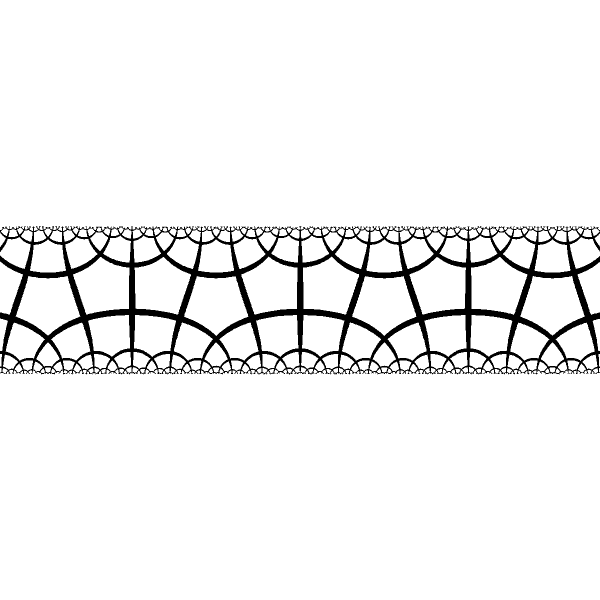Period varies for different pairs of fixed points.

# Different Periods in the Band Model 8Period varies for different pairs of fixed points.

# Different Periods in the Band Model 9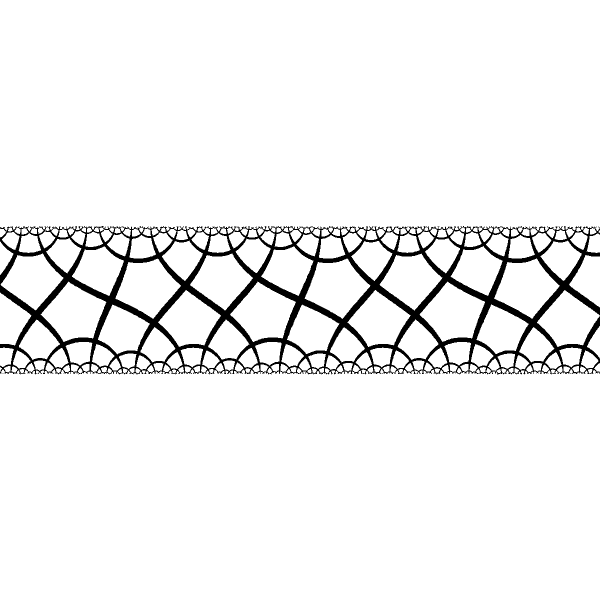Period varies for different pairs of fixed points.

# Different Periods in the Band Model 10Period varies for different pairs of fixed points.

# Different Periods in the Band Model 11Period varies for different pairs of fixed points.

# Different Periods in the Band Model 12Period varies for different pairs of fixed points.

# Different Periods in the Band Model 13Period varies for different pairs of fixed points.

# Mixing Fixed Points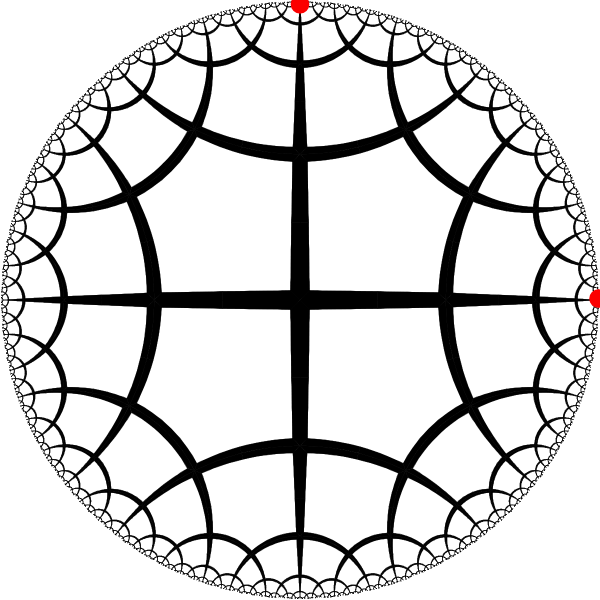We can mix fixed points belonginh to the different isometries

# Move FPs into the Canonical Position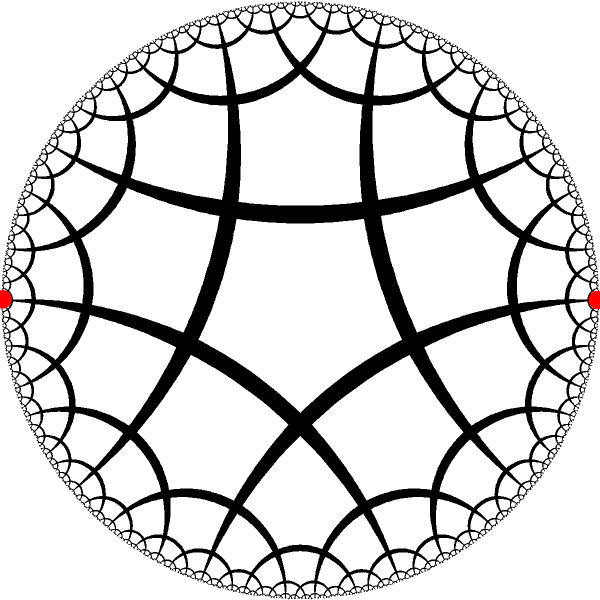We move fixed points into the canonical position: -1, +1

# Stretching Disk into the BandWe stretch the disk into the band. When fixed points are of the same type, both ends of the band have the same asymptotic bevaviour and we have smooth transition between these asymptotes in the center

# Mixing Fixed Points 2When fixed points are of the different type we have different periodical structures at the ends and smooth transition at the center

# Mixing Fixed Points 3When fixed points are of the different type we have different periodical structures at the ends and smooth transition at the center

# Mixing Fixed Points 4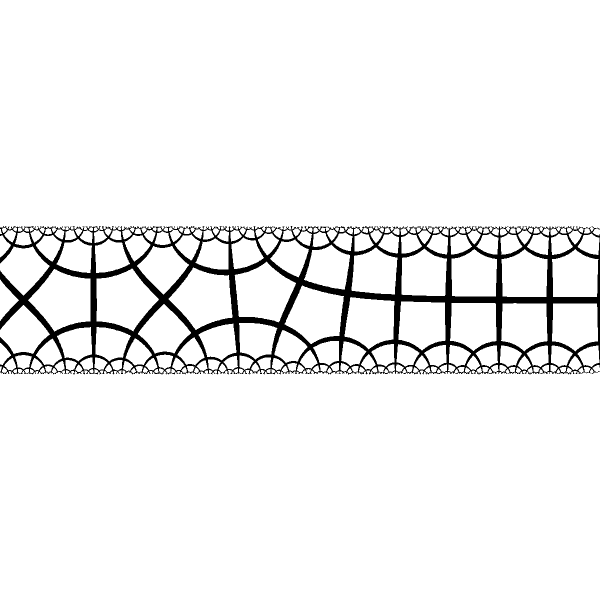When fixed points are of the different type we have different periodical structures at the ends and smooth transition at the center

# Mixing Fixed Points 5When fixed points are of the different type we have different periodical structures at the ends and smooth transition at the center

# Mixing Fixed Points 6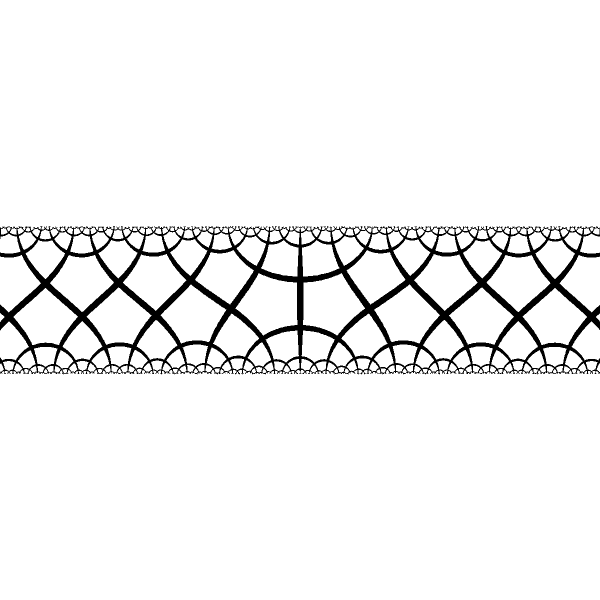When fixed points are of the different type we have different periodical structures at the ends and smooth transition at the center

# Details of the Band Mapping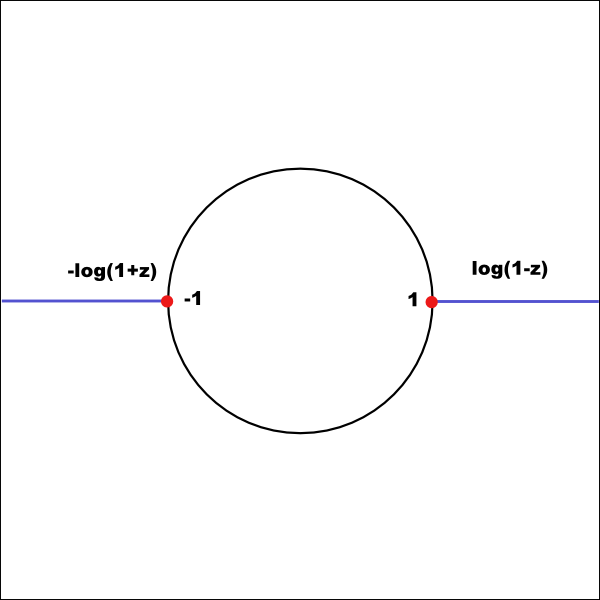Proper way to calculate mapping by tanh^-1 on complex plane is to use linear combination of log() tanh^-1(z)=(1/2)(\log(1+z)-\log(1-z)) The map has 2 branching points with 2 cuts going from points -1 and 1 at the unit circle to \infty

# Bending the BandWe can move one of the points and the corresponding cut anywhere we want by the following simple modification of the mapping w = (1/2)(-a \log(1-az)-\log(1-z)) This will make a Bended Band Model of the hyperbolic plane. [animation] of the mapping with moving point a on the unit circle

# Generalization - Star ModelWe may have arbitrary number of arms placed at some arbitrary locations w = \sum_{i} b_i \log(1-a_i z) During calculation we have to be carefull about the locations of the cuts and take apropriate branch of the log function.

# Star Model of Hyperbolic Plane 1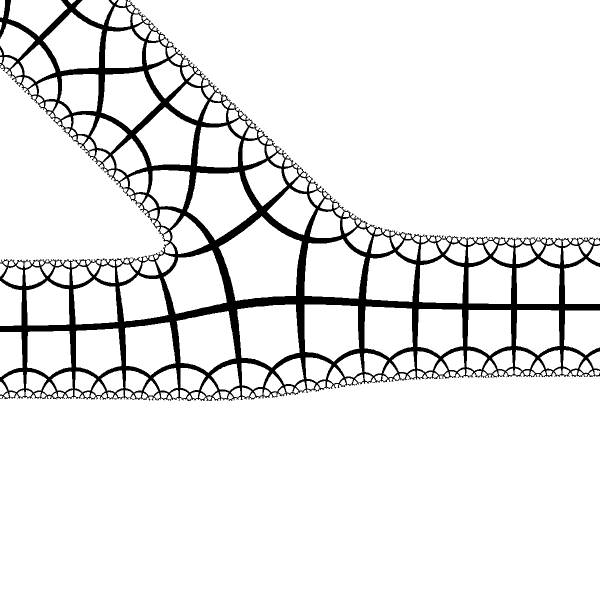Mapping with 3 arms. Two arms are located at the fixed poits of the same type, third arm is at the fixed point of different type.

# Star Model of Hyperbolic Plane 2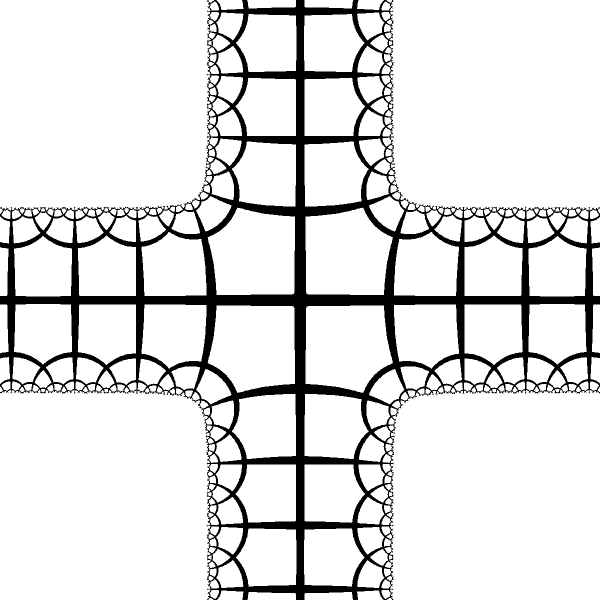Mapping with 4 arms. All arms originate from the fixed points of the same type. Therefore the arms have the same euclidean asymptotic behaviour.

# Star Model of Hyperbolic Plane 3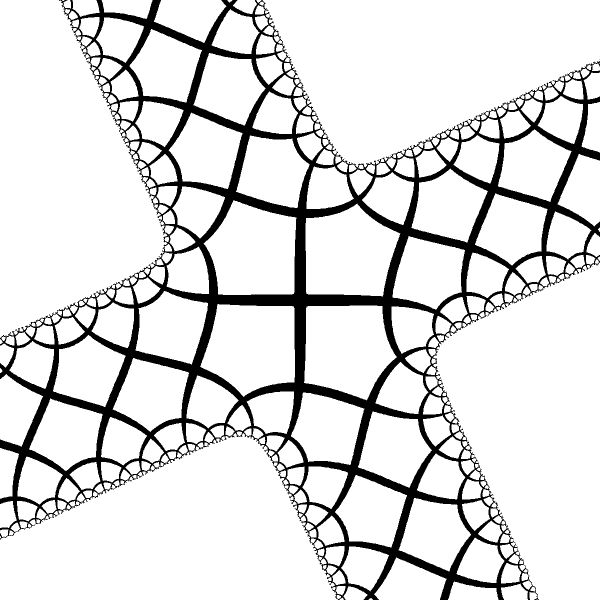Mapping with 4 arms. All 4 fixed point have the same type, but different from the above.

# Star Model of Hyperbolic Plane 4Another 4 arms mapping.

# Star Model of Hyperbolic Plane 5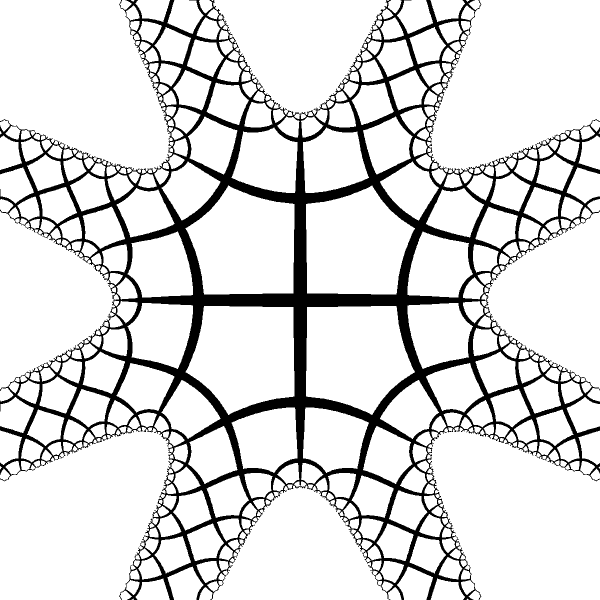Mapping with 8 identical arms.

# Star Model of Hyperbolic Plane 6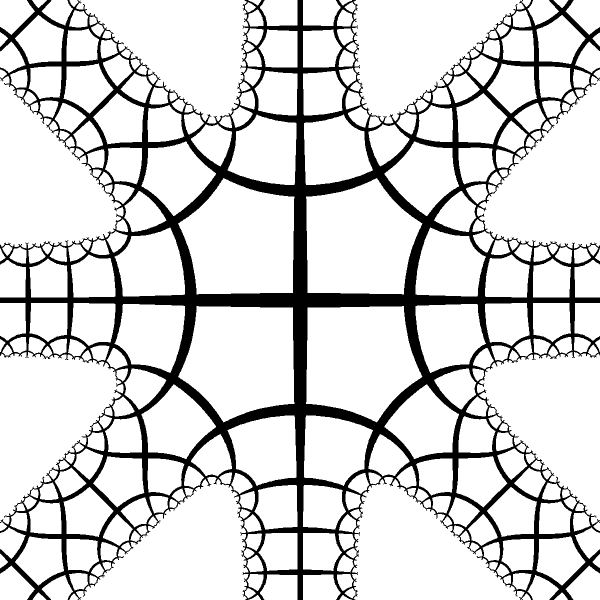Mapping with 8 arms of different type.

# Star Model of Hyperbolic Plane 7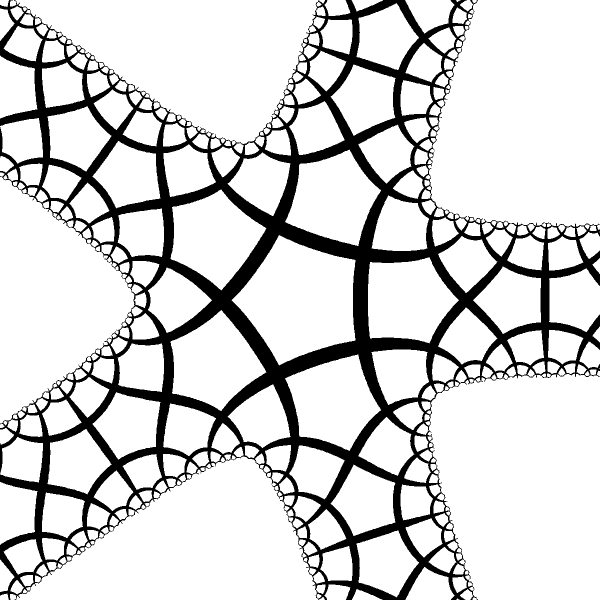We move origin of the hyperbolic plane into the center of pentagonal tile. Now we have 5 symmetrical arms of the same type as in 4 arms case before.# Star Model of Hyperbolic Plane 8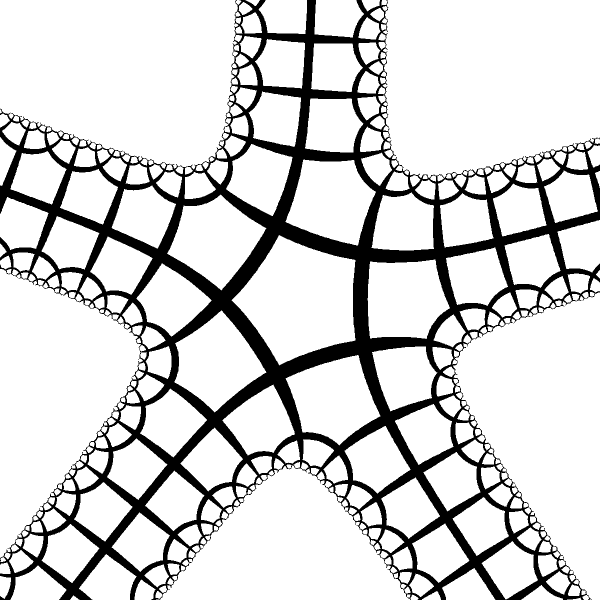Another comparision of 5 and 4 arms stars# Star Model of Hyperbolic Plane 9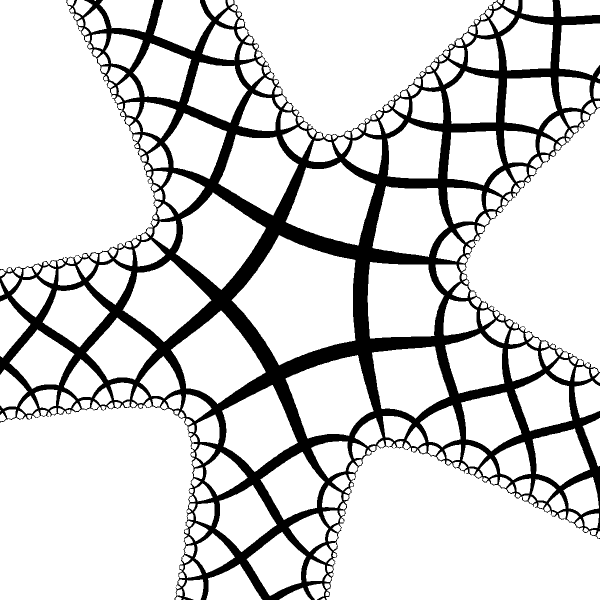Another comparision of 5 and 4 arms stars# Arbitrary Conformal Model

• By the uniformization theorem, any simply connected noncompact Riemann surface other than the whole complex plane CC is a model of the hyperbolic plane.
• According to the Riemann mapping theorem any connected open subset of the complex plane can be mapped to the unit disk. The problem is to find the mapping explicitly.
• Few random and fancy examples follows.

# Disk with CutsDisk with 4 symmetrical cuts [animaton] of hyperbolic isometry in this model.

# Disk to Half Disk + Reflections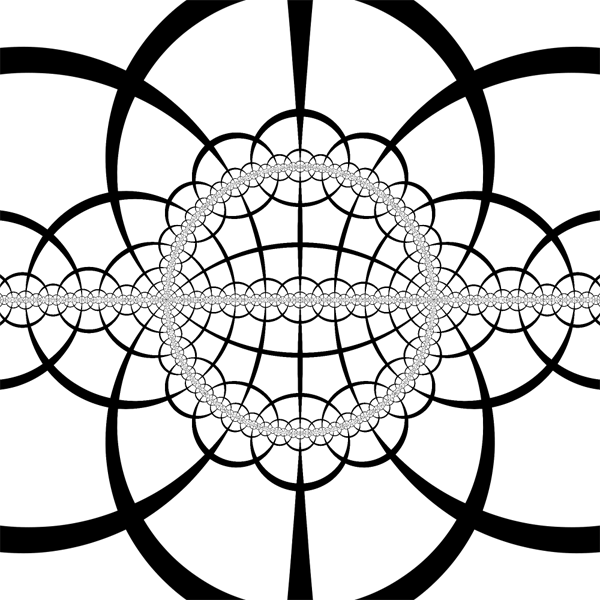Disk is mapped to half disk and 3 reflections in its sides are added

# Disk to Half Disk + ReflectionsHyperbolic disk model is translated, disk is mapped to half disk and 3 reflections in its sides are added.

# Disk to Half Disk + Reflections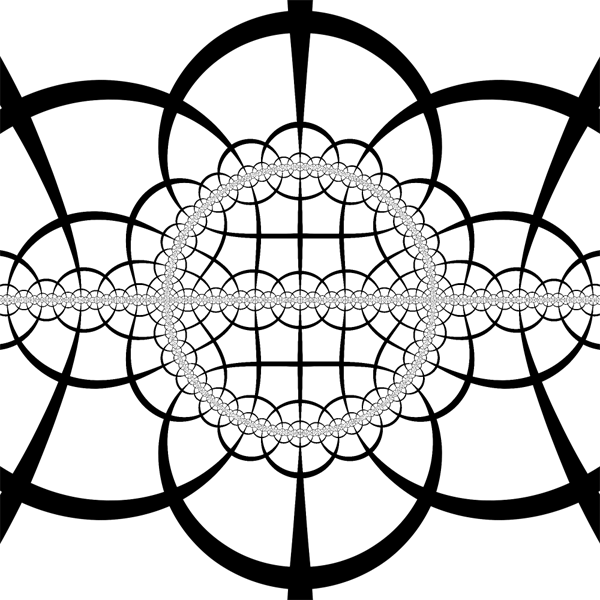Hyperbolic disk model is translated, disk is mapped to half disk and 3 reflections in its sides are added.

# Outside of the Julia Set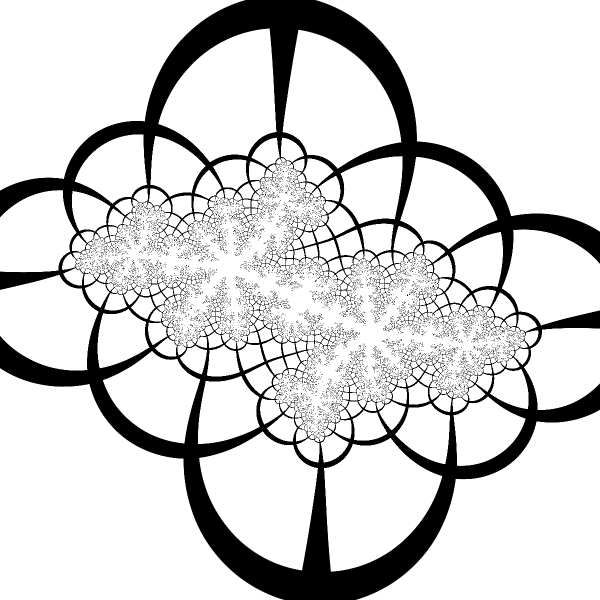Poincare disk is mapped to outside of the unit disk. Outside of the unit disk is mapped to the outside of the Julia set of quadratic map z \to z^2+c. [animation] of the hyperbolic motion in the Julia or Fractal model of the hyperbolic plane

# Symmetry 433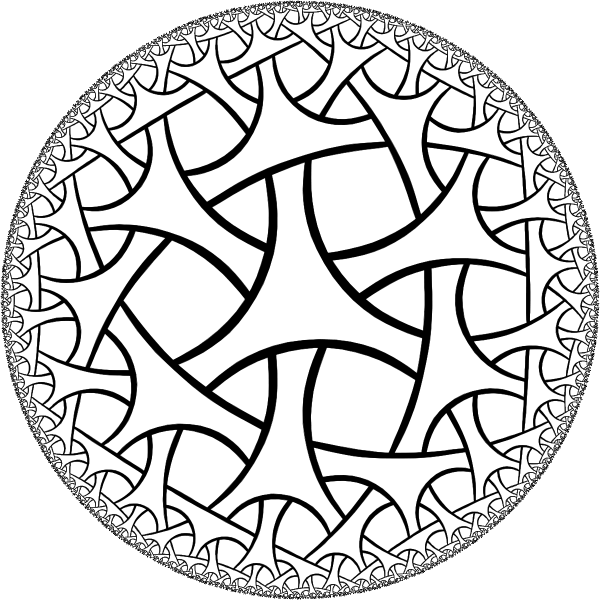A 443 pattern in the hyperbolic plane in the disk model

# Symmetry 433 #2The same patterns in the band model. One curve became straight line. This mean, that all the curves of this tiling are in fact equidistant lines.

# Symmetry 433 #3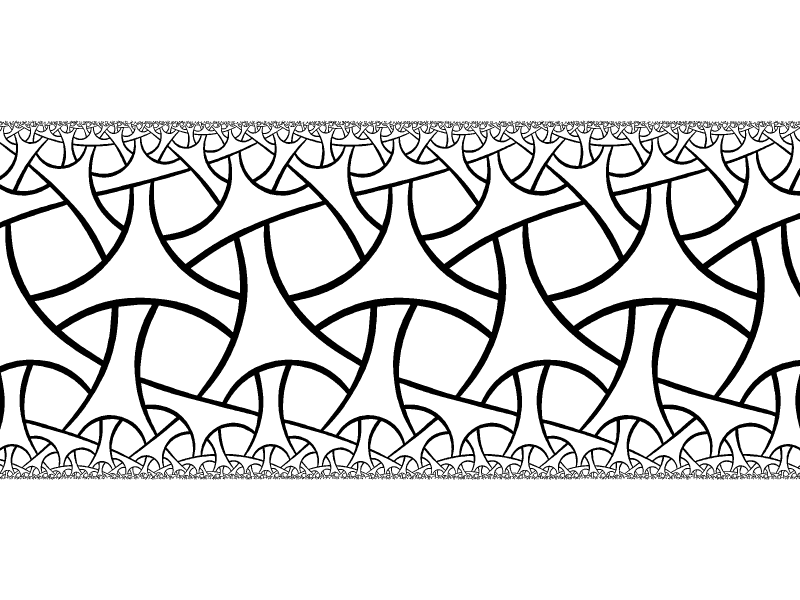Another view of the same patterns

# Symmetry 433 #4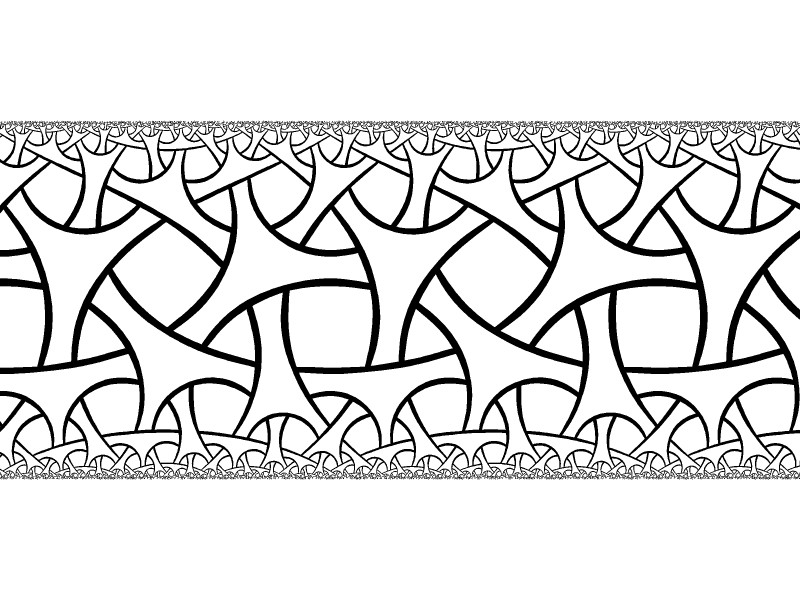Yet another view of the same pattern

# Symmetry 433 #5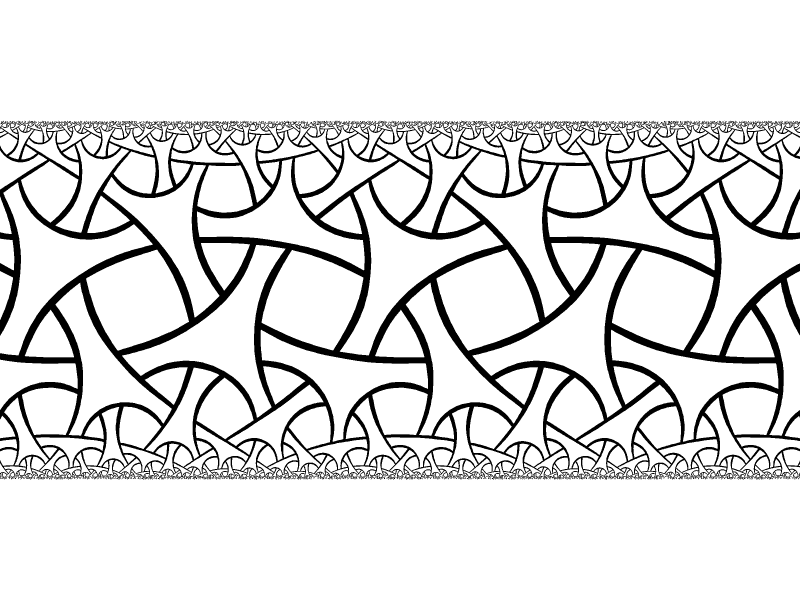The last view of the same pattern

# Symmetry 23X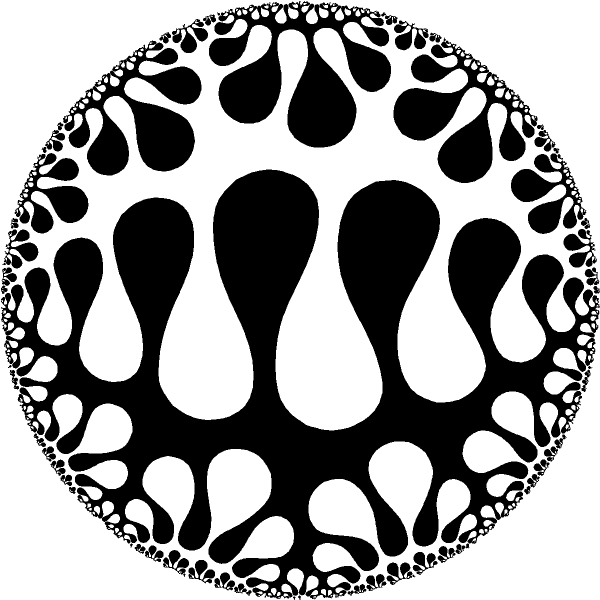23x tiling with 2 colors in the disk model

# Symmetry 23X #2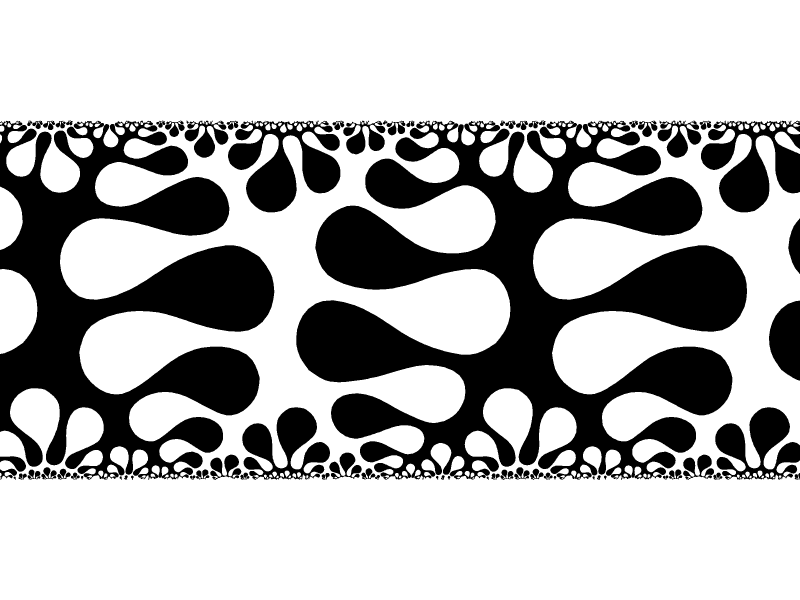The same 23x tiling with 2 colors in the band model

# Symmetry 23X #2Another view of the same 23x tiling

# Symmetry 23X #3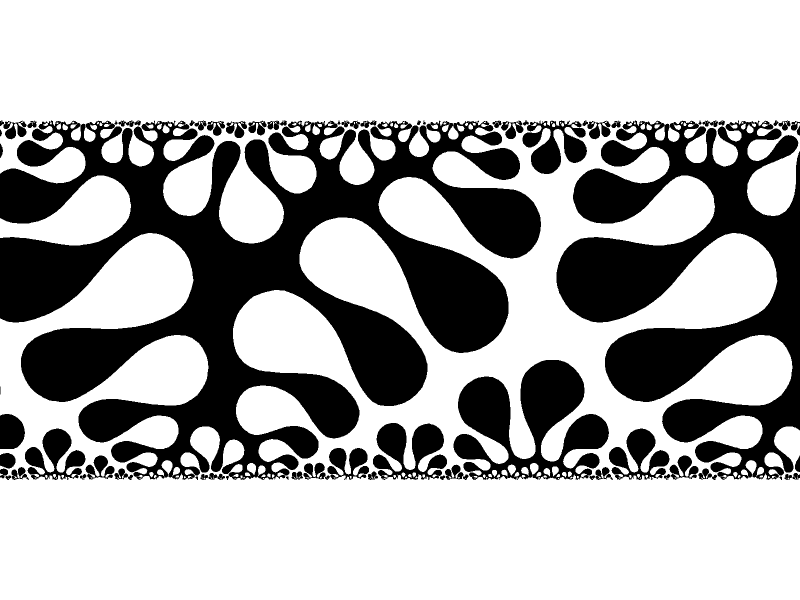Last view of the same 23x tiling

# Sector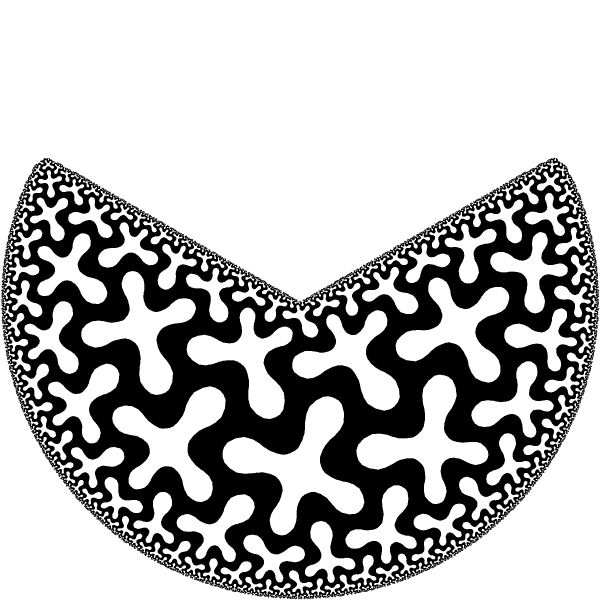4\star 3 Tiling of the sector.

# Disk with a CutTiling of the disk with one cut. It is a sector with angle 360^o - not the same as the unit disk.

# RingTiling of the a ring. Ring can be obtained from the band model using w = exp(z/a) with appropriate a \in R to make mapping periodic around the ring.

# Brooch?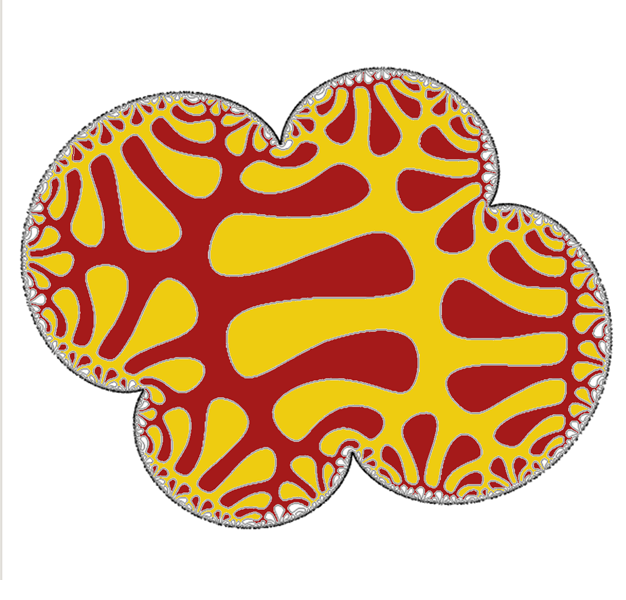Some polynomial mapping of the disk. May be I will convert it into a piece of jewelry someday.

# Polygon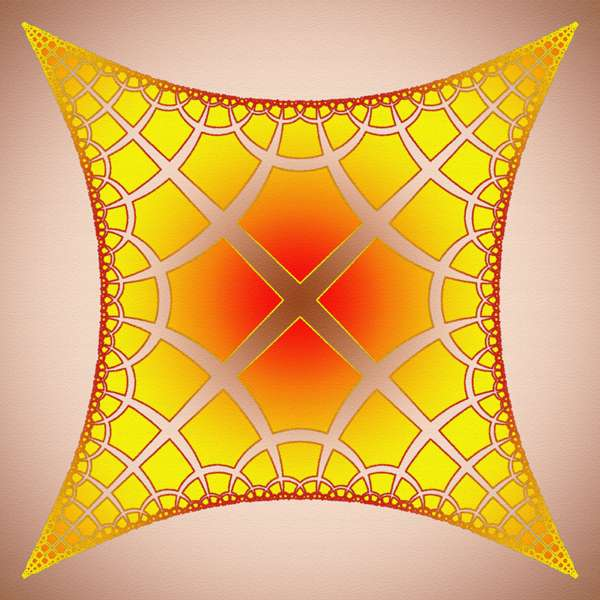Mapping of the disk onto (almost) polygonal area

# Stairs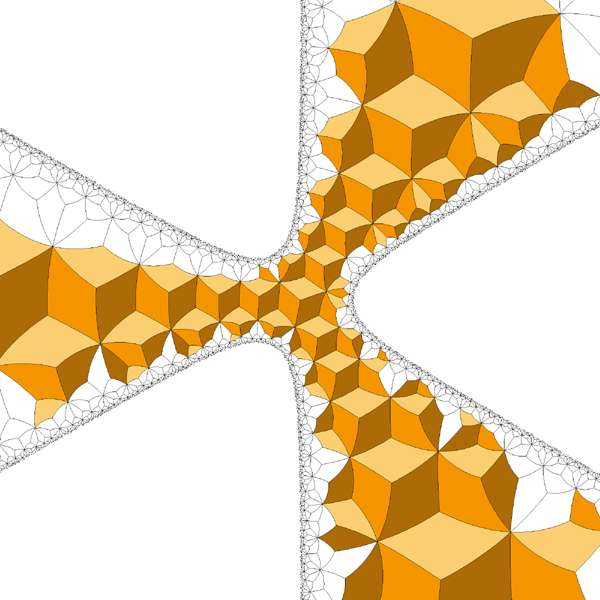I have no idea how I've got this.

# Star23x tiling of star with with 6 identical arms

# M.C.Escher Circle Limit IIITiling of Poicare circle derived from M.C.Escher "Circle Limit III" Escher have made it without math and computer. Only compas, straight edge and steady hand. Amazing!

# M.C.Escher Circle Limit III #2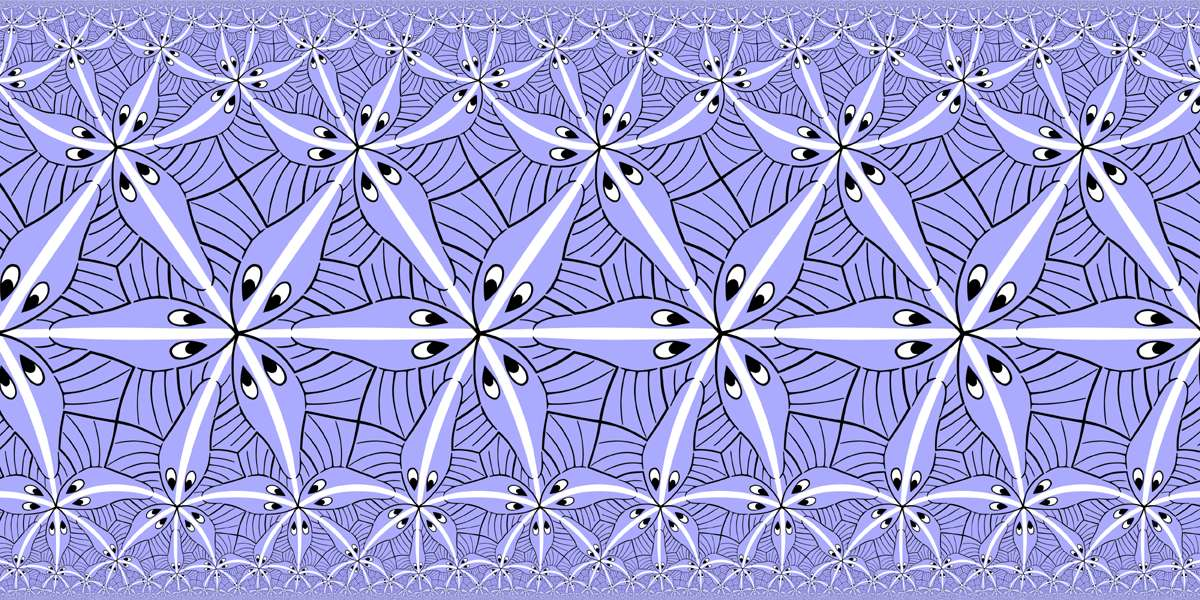The same tiling in the band model

# M.C.Escher Circle Limit III #3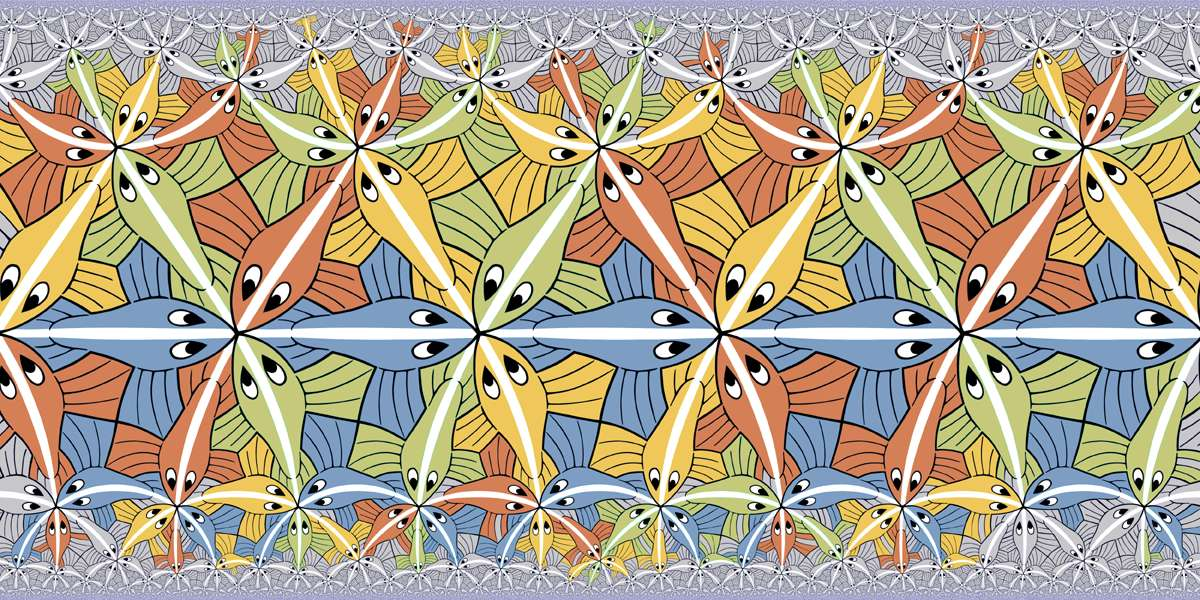The same tiling colored with 4 colors

# M.C.Escher Circle Limit III #4Another version of the colored tiling

# Generalization for H3 - Cylinder ModelStraightforward generalization of the log(1-z) mapping to 3D will transform Poincare ball into an infinite cylinder. It can be called Cylinder Model of H3. Here we have tiling of H3 with right angled dodecahedra (only central chain is shown). Cylinder model is not conformal, but quasi conformal with anisotropy factor close to 1 (\pi/2?) Multi armed 3D stars are also possible.

# Conclusion

The presented conformal models may be used as a visualization tools for hyperbolic geometry. New way of visualization of specific concept helps to create better intuitive understanding of the concent and to make new discoveries.

Conformal mappings of hyperbolic plane seems to show nice properties of the geometry - there is a lot of hyperbolic plane squeezed near the boundary of the Poicare disk and various conformal mappings unwrap this parts of the plane into the visible existence.

Different mappings of the same hyperbolic pattern provide strikingly different views, which higlights different properties of the pattern.

### References

There are a lot of examples of visualization of the hyperbolic geometry in the disk and upper half plane models. First one were made by Klein and Fricke in Vorlesungen uber die Theorie der elliptischen Modulfunktionen, 1890. Check it, it is good.
As far as I know the band model was mentioned without illustrations in exercises in
Milnor J. - Dynamics in One Complex Variable, 1993. It is described with a few nice illustrations in Hubbard J. - Teichmuller Theory. Vol.1, 2008.
First time I have seen the hyperbolic tiling in the band model was in private communication from Anton Sherwood in the summer of 2008. He has a nice example on his web page where he calls it 'Mercator Projection'.